1. /
2. CBSE
3. /
4. Class 10
5. /
6. Mathematics
7. /
8. CBSE Question Paper 2015...

# CBSE Question Paper 2015 class 10 Mathematics### myCBSEguide App

Download the app to get CBSE Sample Papers 2023-24, NCERT Solutions (Revised), Most Important Questions, Previous Year Question Bank, Mock Tests, and Detailed Notes.

CBSE Question Paper 2015 class 10 Mathematics conducted by Central Board of Secondary Education, New Delhi in the month of March 2015. CBSE previous year question papers with solution are available in myCBSEguide mobile app and cbse guide website. The Best CBSE App for students and teachers is myCBSEguide which provides complete study material and practice papers to cbse schools in India and abroad.

Question Paper 2015 class 10 Mathematics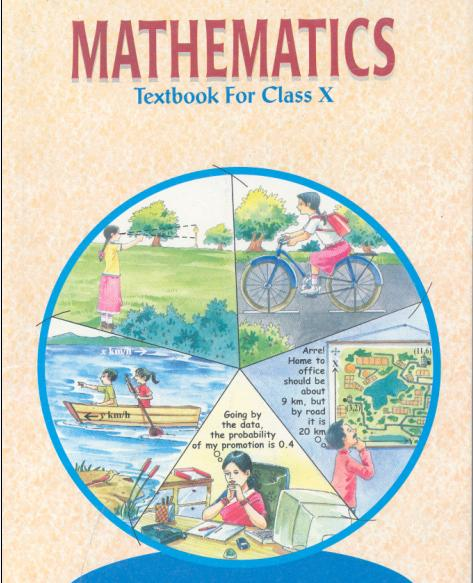## Class 10 Mathematics list of Chapters

1. Real Number
2. Polynomials
3. Pair of Linear Equations in Two Variables
5. Arithmetic Progressions
6. Triangles
7. Coordinate Geometry
8. Introduction to Trigonometry and its Applications
9. Circles
10. Constructions
11. Area Related to Circles
12. Surface Area and Volumes
13. Statistics and Probability

## Last Year Question Paper Class 10 Mathematics 2015

General Instructions:

a. This question paper contains four parts A, B, C, and D.
b. All questions are compulsory for all.
Section-A comprises of 4 questions of 1 mark each, Section-B comprises of 6 questions of 2 marks each, Section-C comprises of 10 questions of 3 marks each and Section-D comprises of 11 questions of 4 marks each.
c. There is no overall choice
d. Use of calculator is not permitted

### Section – A

1. Find sum of 10 terms of following A.P. :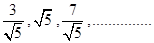2. A tower stands near an airport. The angle of elevation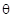of the tower from a point on the ground is such that its tangent is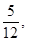find the height of the lower, if the distance of the observer from the tower is 120 meters.

3. A die is thrown once. Find the probability of getting “at most 2.”

4.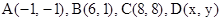are the four vertices of a rhombus taken in order. Find the co-ordinates of point D.

### Section – B

5. Ram Prasad saved 10 in the first week of a year and then increased his weekly savings by 2.75. If in the nth week, his savings become `59.50, find n.

6. Find the roots of the quadratic equation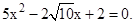7. Two tangents PA and PB are drawn to the circle with centre O such that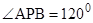Prove that OA=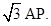8. Draw a circle of radius 3.6 cm. Take a point P outside the circle and construct a pair of tangents to the circle from that point.

9. In two concentric circles, a chord of length 24 cm of larger circle becomes a tangent to the smaller circle whose radius is 5 cm. Find the radius of the larger circle.

10. In the given figure, OAPB is a sector of a circle of radius 3.5 cm with the centre at O. If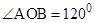, then find the length of OAPBO. (use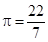)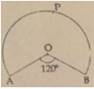### Section – C

11. Find the sum of n terms of the sequence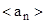where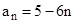and n is a natural number.

12. The sum of a number and its reciprocal is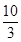, find the number.

13. Draw a circle of radius 3 cm. Construct two tangents at the extreme ties of a diameter of this circle.

14. A man observes the angle of elevation of a bird to be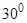. He then walks 100 m towards the birds which is stationary and finds that the angle of elevation is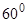. Find the height at which the bird is sitting.

15. From a well shuffled pack of 52 cards, two black queens and two kings are removed. From the remaining cards, a card is drawn at random. What is the probability that drawn card is :
a) a face card
b) an ace

16. Show that the line-segments joining the points (4, 2) and (-6,4) and (-10, 5) and (8, 1) bisect each other.

17. The coordinates of the vertices of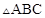are A( 7, 2), B (9,10) and C(1, 4). If E and F are the mid points of AB and AC respectively, prove that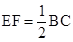.

18. In a cylinder of base radius 10 cm, liquid is filled to the height of 9 cm. A metal cube of diagonal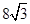cm is immersed completely in the liquid. Find the height by which the water will rise in the cylinder.

19. The wheel of a motor cycle is of radius 21 cm. How many revolutions per minute must the wheel make so as to keep a speed of 77 km/h?

20. A solid metallic sphere of radius 10.5 cm is melted and recast into a number of smaller solid cones, each of radius 3.5 cm and height 3 cm. Find the number of cones so formed.

### SECTION – D

21. If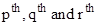term of an A. P. are a, b and c respectively, then show that: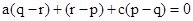22. Solve: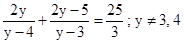23. If the equation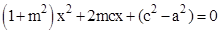has equal roots, prove that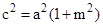24. In the figure FG is a tangent to the circle with centre A. If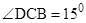and CE=DE, find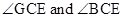.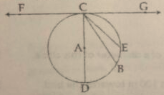25. Draw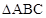such that BC=5 cm,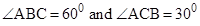, now construct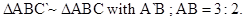26. Two pillars of equal heights stand on either side of a road, which is 200 m wide. The angles of elevation of the top of the pillars are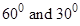at a point on the road between the pillars. Find the position of the point between the pillars and height of each pillar.

27. 17 cards numbered 1, 2, 3 ……… , 16, 17 are put a box and mixed thoroughly . One person draws a card from the box. Find the probability that the number on the card is
(a) odd
(b) a prime
(c) divisible by 3.
(d) divisible by 3 and 2 both.

### 28. Prove that the points A(0, 0), B( 0, 2 ) C (2, 0) are the vertices of an isosceles right triangle. Also, find its area.

29. If h, C and V respectively represent the height, curved surface area and volume of a cone, prove that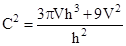30. The area of equilateral triangle is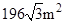. Three circles are drawn at the vertices of the triangle with radius equal to the half of side of triangle. Find the area of the triangle not included in the sectors.

31. A school thought to collect the rainwater from the roof of the building, whose dimensions are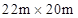by draining into a cylindrical vessel having diameter 7 m and height 4.2 m. If the vessel is just full, find the rainfall recorded in cm.

Why it is necessary to conserve water by doing, these type of activities?

These are questions only. To view and download complete question paper with solution install myCBSEguide App from google play store or login to our student dashboard.

## CBSE Question Paper 2015 class 10 Mathematics

Download class 10 Mathematics question paper with solution from best CBSE App the myCBSEguide. CBSE class 10 Mathematics paper 2015 in PDF format with solution will help you to understand the latest question paper pattern and marking scheme of the CBSE board examination. You will get to know the difficulty level of the question paper.

## Previous Year Question Paper for class 10 in PDF

question papers 2019, 2018, 2017, 2016, 2015, 2014, 2013, 2012, 2011, 2010, 2009, 2008, 2007, 2006, 2005 and so on for all the subjects are available under this download link. Practicing real question paper certainly helps students to get confidence and improve performance in weak areas.

To download CBSE Question Paper class 10 Mathematics, Science, Social Science, English Communicative, English Language and Literature, Hindi Course A, Hindi Course B, and Other subjects; do check myCBSEguide app or website. myCBSEguide provides sample papers with solution, test papers for chapter-wise practice, NCERT solutions, NCERT Exemplar solutions, quick revision notes for ready reference, CBSE guess papers and CBSE important question papers. Sample Paper all are made available through the best app for CBSE students and myCBSEguide website.Test Generator

Create question paper PDF and online tests with your own name & logo in minutes.myCBSEguide

Question Bank, Mock Tests, Exam Papers, NCERT Solutions, Sample Papers, Notes

### 1 thought on “CBSE Question Paper 2015 class 10 Mathematics”

1. I am Umansh Patel
I study in class 10th
Please send in my email 2015to 2023 to all previous year question paper